# Working with Formula column in Dataverse for Teams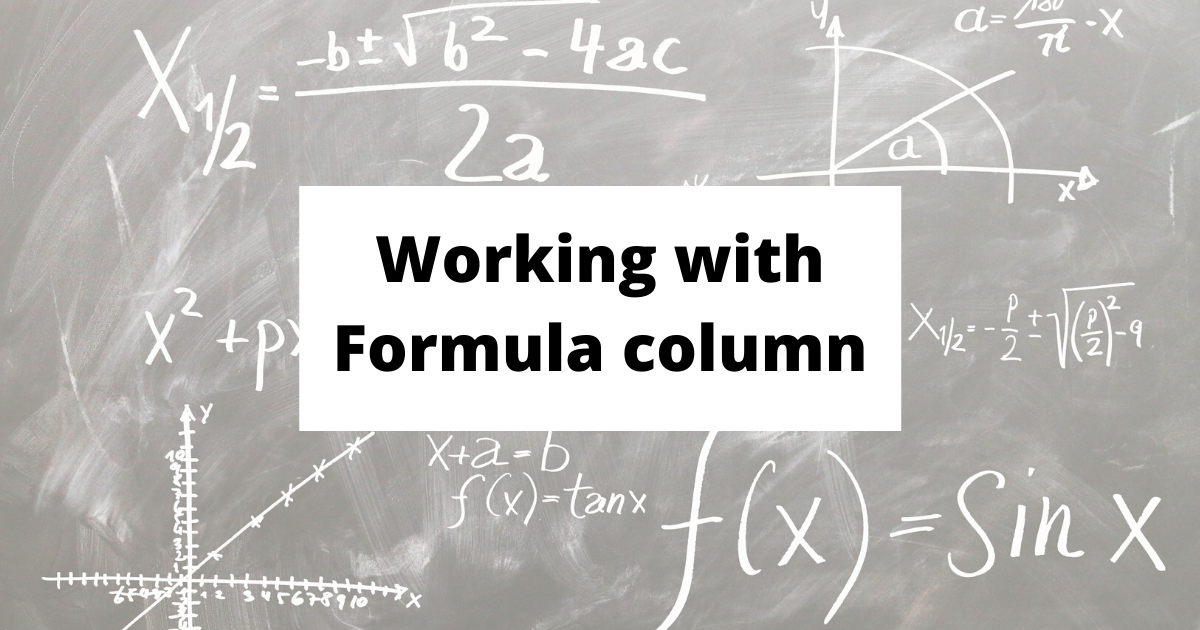# Overview

Formula column is one of data type in Dataverse for Teams leveraging Power Fx. You can add a formula column to table familiar with the Canvas app.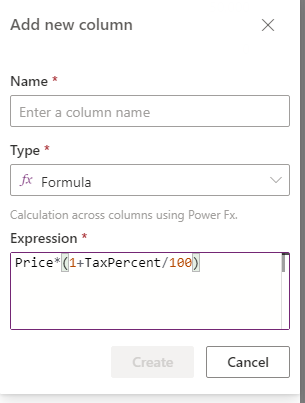In "Expression" box, you can build formula using other columns -Single/Multi line text, Number, Date/DateTime, Choices, LookUp, Part of Currency etc., except for "File" and "Image" type column.

Functions supported in formula column is very limited rather than usual Canvas app formula. (Available functions are listed in Docs)

Expression has to be "One-liner code", you can not use "Set" variables in expression and not "With" to define parameters used for subsequent formula.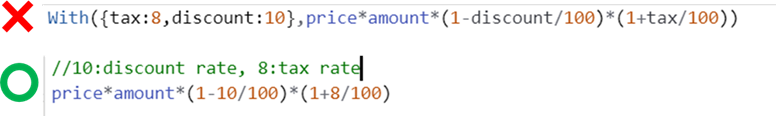# Formula columns in Canvas app

Since formula column is auto-calculated by server, maker can not patch data to that column and form field is locked as "View" only mode reflecting calculated column nature.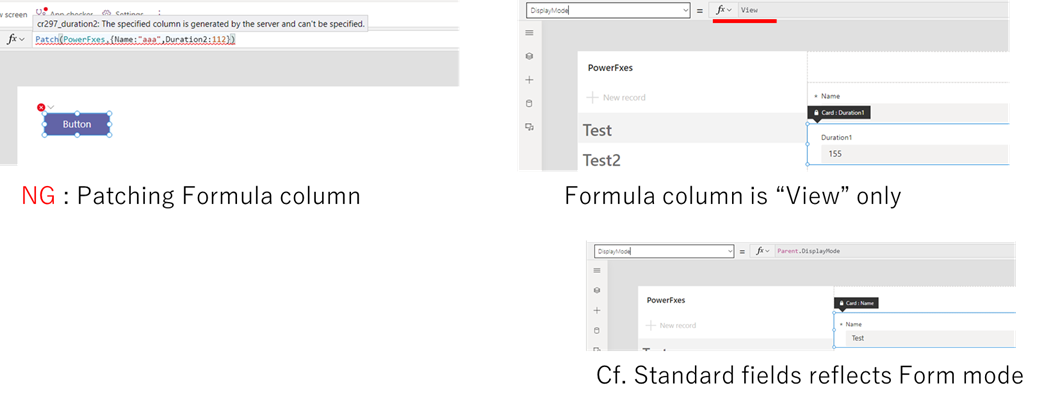# Tips 1: Comment in expression box

To make expression readable for other makers, you can put comment in expression box.

Both single-line and multi-line comments are supported and saved in column setting.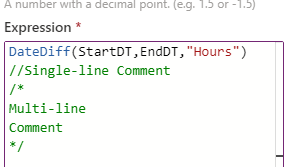# Tips 2: Formula column using formula columns

Formula columns can also refer to another formula column in its expression, as well as other regular type columns.

If you have to define a complex expression, you may define the middle expression with other formula columns and use them.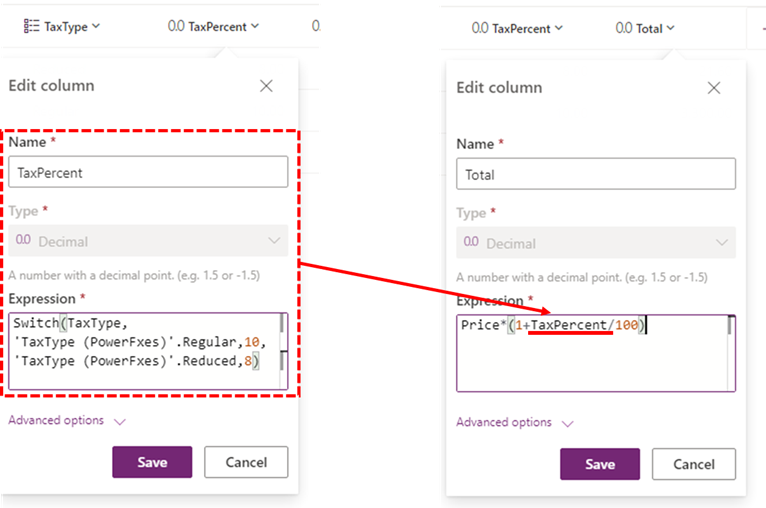That's all!